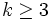# Nilpotent not implies abelian

This article gives the statement and possibly, proof, of a non-implication relation between two group properties. That is, it states that every group satisfying the first group property (i.e., nilpotent group) need not satisfy the second group property (i.e., abelian group)
View a complete list of group property non-implications | View a complete list of group property implications

## Statement

A nilpotent group need not always be abelian.

## Proof

The smallest examples of nilpotent non-abelian groups are of order 8 (see groups of order 8):

• quaternion group, an eight-element group whose elements are$\pm 1, \pm i, \pm j, \pm k$ with the multiplication given by multiplication of quaternions.
• dihedral group of order eight, generated by an element of order four and an element of order two that conjugates the element of order four to its inverse.

## Related facts

### Converse

The converse is true: abelian implies nilpotent.

### Reduction to prime power case for finite groups

Any group of prime power order is nilpotent, so any such group that is non-abelian furnishes an example of a nilpotent non-abelian group.

In a sense, the examples of prime power order are representative of all examples for finite groups, because of the following: By the equivalence of definitions of finite nilpotent group, a nilpotent group is the direct product of its Sylow subgroups. Thus, for a nilpotent group, if the group is an A-group (all Sylow subgroups are abelian) then it must be an abelian group. Thus, any example of a nilpotent non-abelian group ultimately relies on an example of prime power order.

## Related specific information

### Numerical comparisons of number of abelian and nilpotent groups for prime power orders

Order Information on groups Number of abelian groups Number of nilpotent groups Comment$p^k$,$p$ prime groups of prime power order number of unordered integer partitions of$k$, independent of$p$, roughly exponential in$\sqrt{k}$ roughly exponential in$k^3$, but base of exponent depends on$p$. See Higman-Sims asymptotic formula on number of groups of prime power order The counts are substantially different as$k \to \infty$ for fixed$p$. For$k \ge 3$, the counts are different but both are eventually constant as$p \to \infty$ for$k = 3,4$. For$k \ge 5$, the count for abelian groups remains fixed as$p \to \infty$ but the total count grows (roughly) polynomially in$p$, where the degree of the polynomial depends on$k$.$p^3$,$p$ prime groups of prime-cube order 3 5 The two nilpotent non-abelian groups are dihedral group:D8 and quaternion group in case$p = 2$, and prime-cube order group:U(3,p) and semidirect product of cyclic group of prime-square order and cyclic group of prime order for$p$ odd.$p^4$,$p$ prime groups of prime-fourth order 5 14 ($p = 2$), 15 ($p$ odd) There are 6 groups of nilpotency class exactly two and 3 or 4 groups of nilpotency class three.$p^5$,$p$ prime groups of prime-fifth order 7 at least 51 (exact number depends on$p$, grows linearly in$p$) There are groups of nilpotency class values 2, 3, 4$p^6$,$p$ prime groups of prime-sixth order 11 at least 267 (exact number depends on$p$, grows as$\Theta(p^2)$) There are groups of nilpotency class values 2, 3, 4, 5$p^7$,$p$ prime groups of prime-seventh order 15 at least 2328 (exact number depends on$p$, grows as$\Theta(p^5)$) There are groups of nilpotency class values 2, 3, 4, 5, 6

### Numerical comparison of number of abelian and nilpotent groups for finite orders

For any finite number$n$, we have Number of nilpotent groups equals product of number of groups of order each maximal prime power divisor. Similarly, the number of abelian groups is the product over all maximal prime power divisors of the number of unordered integer partitions of the exponent of each.

Thus, for any natural number$n$ such that there is at least one prime number$p$ for which$p^3$ divides$n$, there are nilpotent non-abelian groups of order$n$. For instance, for$n = 144 = 2^4 \cdot 3^2$, there are$5 \times 2 = 10$ abelian groups of that order, and$14 \times 2 = 28$ nilpotent groups of that order. See groups of order 144.